The mid-points of the sides of a triangle ABC are given by (-2, 3, 5), (4, -1, 7) and (6, 5, 3). Find the coordinates of A, B and C.

Asked by Sakshi | 9 months ago |  94

##### Solution :-

Given:

The mid-points of the sides of a triangle ABC is given as (-2, 3, 5), (4, -1, 7) and (6, 5, 3).

By using the section formula,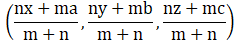We know the mid-point divides side in the ratio of 1:1.

The coordinates of C is given by,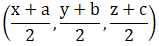P(-2, 3, 5) is mid-point of A(x1, y1, z1) and B(x2, y2, z2)

Then,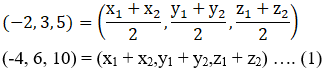Q(4, -1, 7) is mid-point of B(x2, y2, z2) and C(x3, y3, z3)

Then,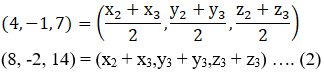R(6, 5, 3) is mid-point of A(x1, y1, z1) and C(x3, y3, z3)

Then,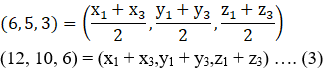Now solving for ‘x’ terms

x1 + x2 = -4……………………(4)

x2 + x3 = 8………………………(5)

x1 + x3 = 12……………………(6)

By adding equation (4), (5), (6)

x1 + x2 + x2 + x3 + x1 + x3 = 8 + 12 – 4

2x1 + 2x2 + 2x3 = 16

2(x1 + x2 + x3) = 16

x1 + x2 + x3 = 8………………………(7)

Now, subtract equation (4), (5) and (6) from equation (7) separately:

x1 + x2 + x3 – x1 – x2 = 8 – (-4)

x3 = 12

x1 + x2 + x3 – x2 – x3 = 8 – 8

x1 = 0

x1 + x2 + x3 – x1 – x3 = 8 – 12

x2 = -4

Now solving for ‘y’ terms

y1 + y2 = 6……………………(8)

y2 + y3 = -2……………………(9)

y1 + y3 = 10……………………(10)

By adding equation (8), (9) and (10) we get,

y1 + y2 + y2 + y3 + y1 + y3 = 10 + 6 – 2

2y1 + 2y2 + 2y3 = 14

2(y1 + y2 + y3) = 14

y1 + y2 + y3 = 7………………………(11)

Now, subtract equation (8), (9) and (10) from equation (11) separately:

y1 + y2 + y3 – y1 – y2 = 7 – 6

y3 = 1

y1 + y2 + y3 – y2 – y3 = 7 – (-2)

y1 = 9

y1 + y2 + y3 – y1 – y3 = 7 – 10

y2 = -3

Now solving for ‘z’ terms

z1 + z2 = 10……………………(12)

z2 + z3 = 14……………………(13)

z1 + z3 = 6……………………(14)

By adding equation (12), (13) and (14) we get,

z1 + z2 + z2 + z3 + z1 + z3 = 6 + 14 + 10

2z1 + 2z2 + 2z3 = 30

2(z1 + z2 + z3) = 30

z1 + z2 + z3 = 15………………………(15)

Now, subtract equation (8), (9) and (10) from equation (11) separately:

z1 + z2 + z3 – z1 – z2 = 15 – 10

z3 = 5

z1 + z2 + z3 – z2 – z3 = 15 – 14

z1 = 1

z1 + z2 + z3 – z1 – z3 = 15 – 6

z2 = 9

Thevertices of sides of a triangle ABC are A(0, 9, 1) B(-4,-3, 9) and C(12, 1, 5).

Answered by Aaryan | 9 months ago

### Related Questions

#### A(1, 2, 3), B(0, 4, 1), C(-1, -1, -3) are the vertices of a triangle ABC. Find the point in which the bisector of the

A(1, 2, 3), B(0, 4, 1), C(-1, -1, -3) are the vertices of a triangle ABC. Find the point in which the bisector of the angle ∠BAC meets BC.

#### If the points A(3, 2, -4), B(9, 8, -10) and C(5, 4, -6) are collinear, find the ratio in which C divided AB.

If the points A(3, 2, -4), B(9, 8, -10) and C(5, 4, -6) are collinear, find the ratio in which C divided AB.

#### Find the ratio in which the line segment joining the points (2, -1, 3) and (-1, 2, 1) is divided by the plane

Find the ratio in which the line segment joining the points (2, -1, 3) and (-1, 2, 1) is divided by the plane x + y + z = 5.